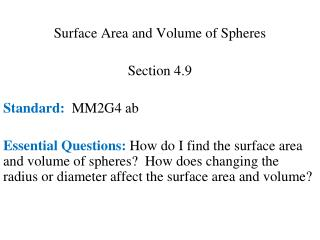DownloadDownload PresentationSurface Area and Volume of Spheres Section 4.9 Standard: MM2G4 ab

# Surface Area and Volume of Spheres Section 4.9 Standard: MM2G4 ab

Télécharger la présentation## Surface Area and Volume of Spheres Section 4.9 Standard: MM2G4 ab

- - - - - - - - - - - - - - - - - - - - - - - - - - - E N D - - - - - - - - - - - - - - - - - - - - - - - - - - -
##### Presentation Transcript

1. Surface Area and Volume of Spheres Section 4.9 Standard: MM2G4 ab Essential Questions: How do I find the surface area and volume of spheres? How does changing the radius or diameter affect the surface area and volume?

2. Formula: Surface Area of a Sphere

3. Find the surface area of the sphere, given that the radius is 9 inches. S = 4(9)2 S = 324 S ≈ 1017.88 in.2

4. 2. Find the surface area of the sphere, given that the diameter is 10 inches. S = 4(5)2 S = 100 S ≈ 314.16 in.2 d = 2r 10 = 2r 5 = r

5. 3. Find the surface area of the sphere, given that the circumference of the sphere is ft. S = 4(4)2 S = 64 C = 2r 8 = 2r 4 = r S ≈ 201.06 ft2

6. Formula: Volume of a Sphere

7. 4. Find the volume of the sphere, given that the radius is 8 inches. V ≈ 2144.66 in.3

8. 5. Find the volume of the sphere, given that the diameter is 10 inches. d = 2r 10 = 2r 5 = r V ≈ 523.60 in.3

9. 6. Find the volume of the sphere, given that the circumference of the sphere is ft. C = 2r 8 = 2r 4 = r V ≈ 268.08 ft3

10. Complete the table. 4 6 4:6 or 2:3 4 6 4:6 or 2:3 4 9 4:9 or 4:9 16 36 16:36 or 4:9

11. Complete the table. 2a 2b a : b 2a 2b a : b  a2  b2 a2 : b2 4a2 4b2 a2 : b2 a3 : b3

12. a : b Scale Factor _____________ Area Ratios _______________ Volume Ratios _______________ a2 : b2 a3 : b3

13. 7. Two spheres have diameters 24 and 36. a. What is the ratio of the areas? b. What is the ratio of the volumes? Ratio of volumes: 24 and 36 Radii: Ratio of radii: 24 : 36 or 2 : 3 Ratio of areas: 22 : 32 or 4 : 9 23 : 33 or 8 : 27

14. 8. A sphere has a radius of 6 meters. The radius of a second sphere is 3 meters. (a.) How does the surface area of the second sphere compare the to surface area of the first sphere? (b.) Volumes? Ratio of radii: 3 : 6 or 1 : 2 or 1: 4 (a). Ratio of areas: 12 : 22 The second sphere is ¼ the size of the first. (The area of the second sphere is 4 times smaller) or 1: 8 13 : 23 (b). Ratio of volumes: The second sphere is 1/8 the size of the first. (The volume of second sphere is 8 times smaller)

15. 9. The radius of a sphere is 2.4 cm. (a.) How will the surface area change if the radius is doubled? (b.) Volume? Double the radius: 2(2.4) = 4.8 Ratio of radii: 2.4 : 4.8 or 1 : 2 12 : 22 or 1 : 4 Ratio of surface areas: The surface area is 4 times larger if the radius is doubled. Ratio of volumes: 13 : 23 or 1 : 8 The volume is 8 times larger if the radius is doubled.

16. 10. Find the surface area of one hemisphere of a circle if the circumference of a great circle of the sphere is 7 cm. C = 2r 7 = 2r 7/2 = r Area of base of hemisphere: A = r2 A =  (3.5)2 A = 12.25 A ≈ 38.48 cm2 Surface area of entire sphere: S = 4r2 S = 4 (3.5)2 S = 49 S ≈ 153.93 cm2 Surface area of hemisphere ≈ 153.93 cm2 + 38.48 cm2 ≈ 192.41 cm2